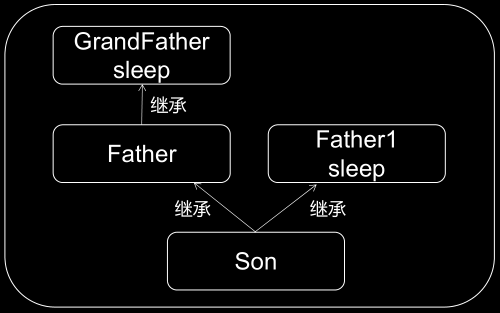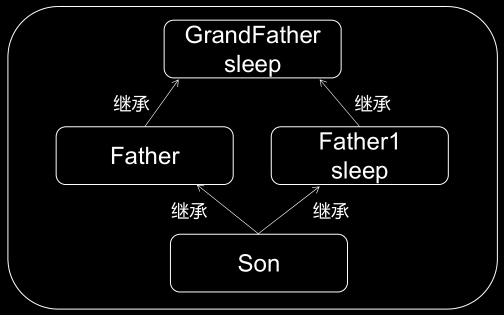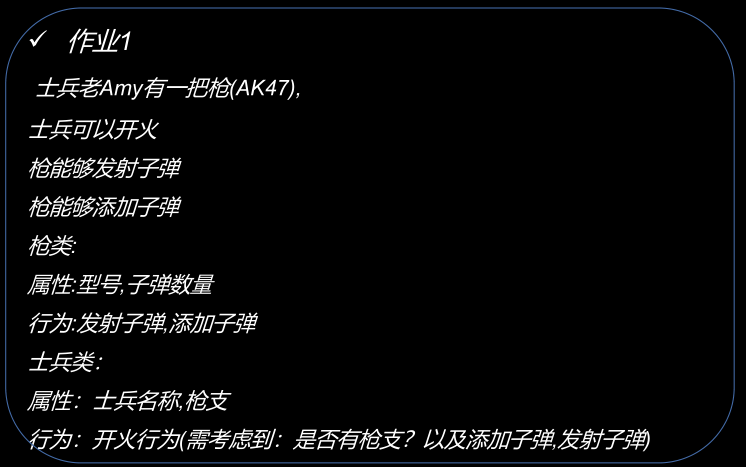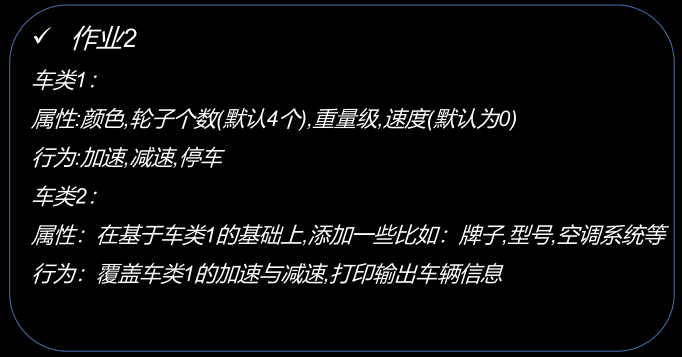# 一木.溪桥学Python-13：多继承、多态、常用魔法方法、单例模式eg. :

class Father(object):
def run(self):
print("Father 会跑")
class Father1(object):
def run(self):
print("Father1 会跑")
class Son(Father, Father1):
pass
s = Son()
s.run()# Father 会跑 ；左边优先eg. :

class GrandFather(object):
def sleep(self):
print("GrandFather 睡觉")
class Father(GrandFather):
def run(self):
print("Father 会跑")
class Father1(object):
def run(self):
print("Father1 会跑")
def sleep(self):
print("Father1 睡觉")
class Son(Father, Father1):
pass
s = Son()
s.sleep() # GrandFather 睡觉; 左边一条路走到底eg. :

class GrandFather(object):
def sleep(self):
print("GrandFather 睡觉")
class Father(GrandFather):
def run(self):
print("Father 会跑")
class Father1(GrandFather):
def run(self):
print("Father1 会跑")
def sleep(self):
print("Father1 睡觉")
class Son(Father, Father1):
pass
s = Son()
s.sleep() # Father1 睡觉 同一个根时，根最后执行
print(Son.__mro__) # .__mro__ 是C3 算法，用于查看继承的执行顺序。
# (<class '__main__.Son'>, <class '__main__.Father'>, <class '__main__.Father1'>, <class '__main__.GrandFather'>, <class 'object'>)

Python 中 用一个函数调用多个不同的功能的函数（具有相同的函数名），实现不同的功能，就形成了多态。

eg. :

class Person(object):
def print_self(self): # 同名函数1，实现不同的功能输出
print("自我介绍")
class Man(Person):
def print_self(self): # 同名函数2，实现不同的功能输出
print("man 的自我介绍")
def print_self(obj): # 下面用这一个函数去调用，同名函数1/2，实现不同的功能输出
obj.print_self() # 调用同名函数.print_self()
zs = Person()
# zs.print_self() # 自我介绍 ,这里是常规的函数调用方法
print_self(zs) # 自我介绍；函数引用，传参zs
hansen = Man()
# hansen.print_self() # man 的自我介绍 ,这里是常规的函数调用方法
print_self(hansen) # man 的自我介绍；函数引用，传参hansen

__doc__ ：用来查看类的说明文档
eg. :
print(list().__doc__)# list() -> new empty list
# list(iterable) -> new list initialized from iterable's items
li = list("abc")
print(li.__doc__)
s = "123"
print(s.__doc__)
__class__ :
__module__:
class Demo(object):
"""

"""
pass
d = Demo()
print(d.__doc__) # 类的说明文本
print(Demo.__module__) # __module__查当前文件所在模块；__main__ ；
print(Demo.__class__) # __class__ 查看对象的类；Demo类是type的对象<class 'type'>
print(d.__class__) # d是Demo的对象<class '__main__.Demo'>
__dict__ ：用于获取类或者实例的属性字典
eg. :
class Demo(object):
country = "中国"
def __init__(self):
self.name = "deng"
self.__age = 18
def test(self):
self.gender = "female"
print("test")
d = Demo()
d.test() # test
print(d.__dict__) # 返回字典，对象去访问，只能访问到对象中的成员（实例属性）
print(Demo.__dict__) # 返回字典，类名去访问，可以访问到类当中的成员（除了实例属性之外的属性与方法）
print(d.__dir__()) # 返回列表，返回所有的成员（__dict__更倾向于是__dir__()的子集）

__del__() ：叫做析构方法。

eg. 1:

class Demo(object):
def __del__(self):
print("我被回收了")
d = Demo()
print("--"*10)
print("--"*10)
# 在所有代码都执行完毕之后，会自动的执行__del__方法
eg. 2:通过关键字 del 将对象删除则会主动的执行 del 方法
class Demo(object):
def __del__(self):
print("我被回收了")
d = Demo()
print("--"*10)
del d # 通过关键字 del 将对象删除则会主动的执行 __del__ 方法
print("--"*10)

Run: -------------------- 我被回收了 --------------------

-eg.3 :只有当对象全部释放 才会自动触发 del

class Demo(object):
def __del__(self):
print("我被回收了")
d = Demo()
d1 = d
print("--"*10)
del d
# del d1# d1 没有被释放，所以 __del__ 还未触发
print("--"*10) # 只有当对象全部释放 才会自动触发 __del__

Run: -------------------- -------------------- 我被回收了

__call__() ：用于将对象变成一个可调用的对象。

class Demo(object):
def __init__(self):
print("aaa")
def __call__(self, *args, **kwargs):
print("我可以调用了奥")
d = Demo()
d() # 未用__call__的话会报错：TypeError: 'Demo' object is not callable
__new__() ：用于创建与返回一个对象。

class Demo(object):
def __init__(self):
print("__init__")
def __new__(cls, *args, **kwargs):
# 重写了父类的new方法，对象并没有创建成功，所以不会再触发__init__方法
print("__new__")
d = Demo()
# 1.__init__创建对象之后自动调用的方法
# 2.__new__用来创建对象的

Run: __new__

__init__() 与 new() 区别： __init__() 实例方法， __new__ 静态方法 __init__() 在对象创建后自动调用， __new__ 创建对象的方法

eg.:

class Demo(object):
def __init__(self):
print("__init__")
def __new__(cls, *args, **kwargs):
print("__new__")
# 重写父类的__new__，还需要执行父类的代码
# super().__new__(cls) # 执行了父类创建对象的代码，还并没有自动的执行__init__
return super().__new__(cls) # 将对象返回出去，自动执行__init__方法
d = Demo()
# Run:
# __new__
# __init__

class Single(object):
__isinstance = None # 1.类属性，建立标识
def __new__(cls, *args, **kwargs):
if cls.__isinstance is None:
cls.__isinstance = super().__new__(cls) # 2.将一个对象存储到__isinstance类属性中。
# 3. 返回 cls.__isinstance, 也就是我们的对象
return cls.__isinstance
else:
# 4. __isinstance 不为None,意味着它已经存储着一个对象，我们直接将返回出去即可。
return cls.__isinstance
s = Single()
s1 = Single()
s2 = Single()
print(id(s)) # 2378210168112
print(id(s1)) # 2378210168112
print(id(s2)) # 2378210168112Run:

Run:

Run:

Run:

Run:End ! Best wishes for you！

## jmeter基础之断言及参数的关联性

jmeter参数关联接口测试中经常会碰到，下一个接口的入参是上个接口的出参，企业中不可能实时进行手工改动，那么就需要用到参数的关联，把接口的响应...